[BACK]
 Computers, Materials & ContinuaDOI:10.32604/cmc.2022.017504Article

A New Task Scheduling Scheme Based on Genetic Algorithm for Edge Computing

1State Grid Information & Telecommunication Group Co., Ltd, Beijing, 102200, China
2University of Chittagong (CU), Chittagong, Bangladesh
*Corresponding Author: Li Wenjing. Email: cara0526@163.com
Received: 01 February 2021; Accepted: 13 July 2021

Abstract: With the continuous evolution of smart grid and global energy interconnection technology, amount of intelligent terminals have been connected to power grid, which can be used for providing resource services as edge nodes. Traditional cloud computing can be used to provide storage services and task computing services in the power grid, but it faces challenges such as resource bottlenecks, time delays, and limited network bandwidth resources. Edge computing is an effective supplement for cloud computing, because it can provide users with local computing services with lower latency. However, because the resources in a single edge node are limited, resource-intensive tasks need to be divided into many subtasks and then assigned to different edge nodes by resource cooperation. Making task scheduling more efficient is an important issue. In this paper, a two-layer resource management scheme is proposed based on the concept of edge computing. In addition, a new task scheduling algorithm named GA-EC(Genetic Algorithm for Edge Computing) is put forth, based on a genetic algorithm, that can dynamically schedule tasks according to different scheduling goals. The simulation shows that the proposed algorithm has a beneficial effect on energy consumption and load balancing, and reduces time delay.

Keywords: Smart grid; energy consumption; task scheduling; resource management

1  Background

With the rapid evolution of smart grid and communication technology , massive amounts of power equipment are now accessing the network, and the number of power service users is increasing day by day. The cloud is capable of storing vast amounts of data and processing computation-intensive tasks with scalability, flexibility, and security [4,5]. Cloud computing can support the power grid through data storage, disaster recovery, intelligent use of electricity, and simulation analysis. The cloud platform can integrate the data and resources of different systems in the grid and manage them, which allows the sharing of data and resources in the smart grid. It is imperative to achieve rational resource management and task scheduling in order to ensure the effectiveness and stable operation of the power cloud computing system.

There has been much research into cloud resource management . In research into saving energy, the authors in  proposed a scheme that can achieve energy savings in a virtual cluster by reducing the processor speed. The authors in  proposed a dynamic resource allocation method that can adjust the number of resource nodes according to actual demand. To reduce the computational complexity of converting low-resolution video to high-resolution while minimizing computation and additional communication costs related to the mobile client, the authors in  investigated video quality enhancement by offloading computing tasks to the mobile cloud computing (MCC) environment.

Researchers focused on increasing the resource utilization rate proposed a two-layer resource scheduling scheme that can achieve rational task scheduling according to the heuristic algorithm [12,13]. The authors in  proposed an energy-saving resource scheduling method that can provide real-time cloud services with a small time delay. In addition, genetic algorithms are used in many areas of cloud computing [15,16], including resource management.

Cloud computing is used for providing resource storage and task computing services in power networks. Because of long transmission delays in cloud computing and limited computing resources in edge nodes , in this paper, we present a two-layer resource management model and a novel task scheduling algorithm to meet the requirements of power networks, as shown in Fig. 1. In smart grid, the edge node can be intelligence terminal, Energy router, concentrators and so on.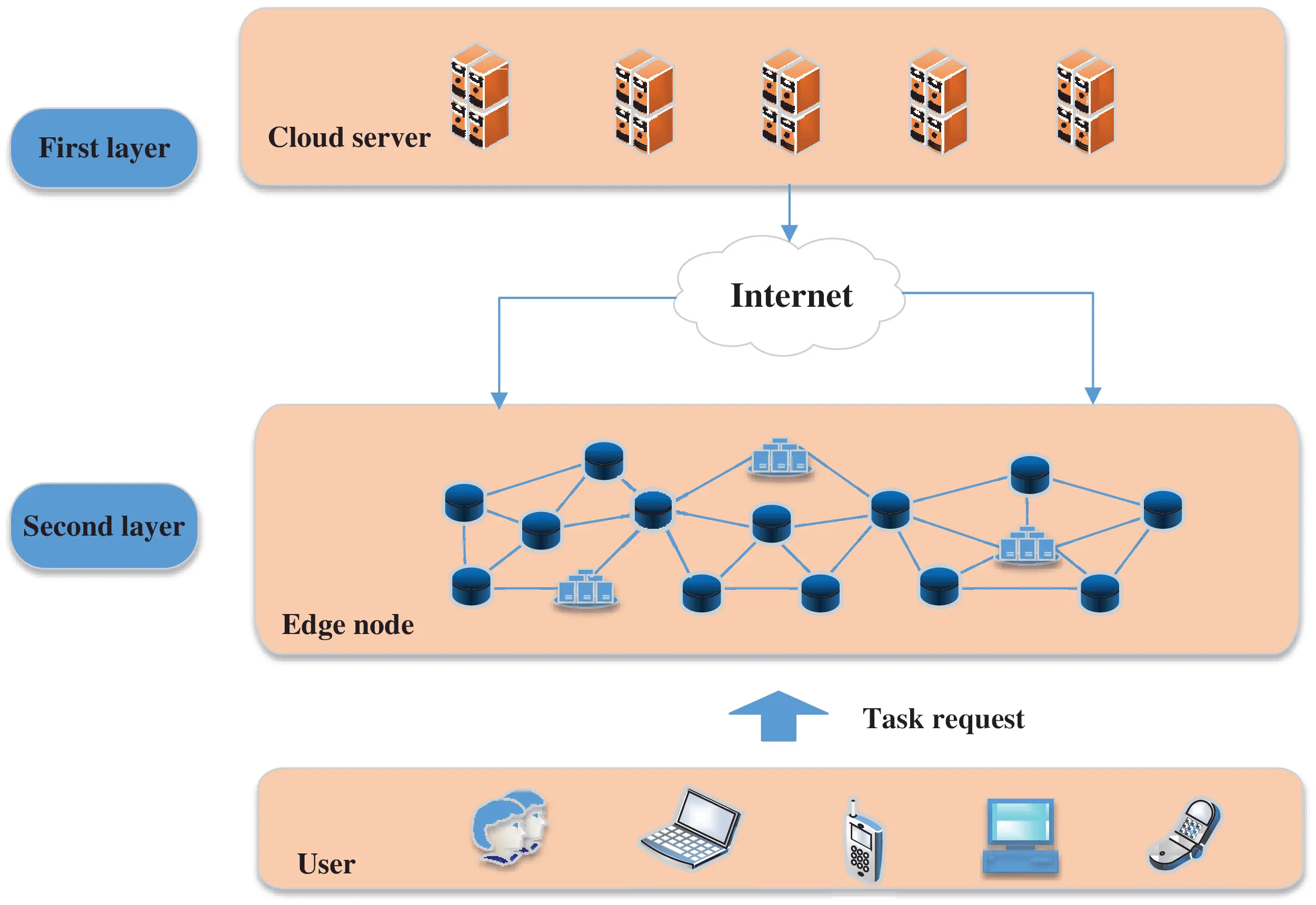Figure 1: The architecture of two-layer resource management model

Hereinafter, the second part introduced the power resource management scheme based on edge computing and the third part in traduced the task scheduling algorithm in detail. In the fourth part, we simulated the performance of the new algorithm with other three contrast algorithms. The fifth part concludes this paper.

2  Power Resource Management Scheme Based on Edge Computing

With the rapid evolution of cloud computing, more and more intelligent terminals are being connected to the network. In traditional cloud computing, all computing tasks are transferred to the cloud data center, which cannot always meet the real-time requirement of users. In addition, bandwidth bottlenecks are inevitable in traditional cloud computing when a large number of computing tasks need to be executed at the same time.

At the edge of a power network, there are many devices, which consume considerable resources . If the resources in these devices can be used for providing computing services, the energy of cloud computing can be expanded. For the resource management model in this paper, some computing tasks in cloud servers can be offloaded to the edge devices, and the local computing tasks can also be performed in the local edge devices.

In the new resource management model, there are two layers for computing nodes. The first layer includes the analysis control nodes that are in the cloud servers, and the second layer includes the computing nodes that are in the edge devices. The first-layer nodes are used for performing computation-intensive tasks and managing the second-layer nodes, which are used for performing local computation tasks. Therefore, the resources in the second-layer nodes should be scheduled uniformly by the first-layer nodes. The resource management model can be used in several application scenarios, such as power supply service command systems, big data platforms, and hot spot locations.

In this resource management model, because the resources of the edge nodes in the second-layer nodes are limited, some tasks will be divided into subtasks that will be performed by different edge nodes. Therefore, the resources in the second layer will be shared and scheduled among different tasks.

3  Introduction of Task Scheduling Algorithm in Detail

The issue of task scheduling in a power network has become increasingly complex. A scheduling algorithm is critical for increasing energy-saving performance, reducing time delay, and improving load balancing. Therefore, in this paper, we propose a novel intelligent optimization algorithm for task scheduling.

For the resource management model proposed in this paper, the edge node is in the second layer, where limited resources affect the completion of some computation-intensive tasks along. Therefore, the computation-intensive tasks will be divided into subtasks and will be performed by different edge nodes. This makes assigning the tasks among the different edge nodes a key issue.

Many scholars have studied task scheduling problems. For example, a task scheduling algorithm called DSFACO was proposed in , which can assign several tasks in the same scheduling queue in order to decrease execution time. This algorithm can improve the running of virtual machine resources. A task scheduling method proposed in  employed a pheromone update rule of the ant colony algorithm by mass function and improved the heuristic information model by loading the standard deviation function to achieve the load balancing of virtual machines in the cloud. A low-power scheduling algorithm called ECMM (which stands for Energy-Considered Min-Min) is presented in , but it was thought that this algorithm might reduce task execution efficiency. The authors in  proposed an energy-saving resource allocation algorithm by migrating the applications with high load to a new multi-core server and migrating the applications with low load to the existing single-core servers.

In the second layer of the resource management model, the computation-intensive tasks will be divided into many subtasks, and the edge nodes will perform the subtasks by cooperating with each other. In this paper, we propose a task scheduling algorithm based on the genetic algorithm to achieve resource sharing and task allocation among the different edge nodes.

3.1 Mathematical Model

In this section, we introduce the computational model and the flowchart of the task scheduling algorithm. For this paper, the number of edge nodes is n, and the number of tasks is m. The set of edge nodes is R={R1,R2…Ri…,Rn}, and the set of tasks is T={T1,T2…Tj…,Tm}. The purpose of task scheduling is to assign the appropriate edge nodes for tasks according to the scheduling objective function.

In the scheduling process between tasks and edge nodes, lij represents the situation where task Tj is assigned to edge node Ri.

lij={1, if Tjisassigned to Rj0, otherwise (1)

In this paper, we consider both the energy consumption for calculation and the energy consumption for transmission. The calculation models are introduced below.

3.1.1 Calculation model of Energy Consumption

For node Ri and task Tj, the calculation time of task Tj on node Ri can be expressed as

CTi,j=li,j×FLi,jCi,j (2)

where FLi,j is the number of CPU cycles requested, and Ci,j is the computational ability of node Ri, which is the number of CPU cycles assigned for task Tj in unit time. Therefore, the energy consumption of node Ri executing task Tj can be expressed as

Ei,jC=PiC×CTi,j (3)

where PjC is the power consumption of computing on node Ri. Therefore, the total energy consumption of node Ri can be introduced as:

EiC=j=1mEi,j (4)

3.1.2 Transmission Model of Energy Consumption

In this section, we will introduce the calculation model for transmission energy consumption. The transmission rate to node Ri can be expressed as

ri,j=Blog2(1+hiUPPiUPBN0) (5)

where B is the channel bandwidth assigned for task Tj, and hiUP is the channel gain between user and node Ri. Furthermore, PiUP is the signal transmission power between user and node Ri by wireless, N0 is the Gaussian white noise. Therefore, the transmission time can be expressed as

TTi,j=TDi,jri,j (6)

where TDi,j is the total data of task Tj assigned on node Ri. Therefore, the energy consumption of node Ri executing task Tj can be expressed as

Ei,jT=TTi,j×Pi,jTrans (7)

where Pi,jTrans is the transmission power between user and node Ri. The total energy consumption of node Ri can be expressed as

EiT=j=1mEi,jT (8)

3.1.3 Objective Function

In this paper, the objective of task scheduling is to decrease energy consumption. The objective function can be expressed as

E=i=1nEi=i=1nEiC+EiT (9)

where E is the total energy consumption of all edge nodes, and the scheduling goal is to reduce the total energy cost of all edge nodes.

3.2 Algorithm Introduction

The specific algorithm flow of task scheduling is based on a genetic algorithm . A genetic algorithm has four main parts: .

3.2.1 Fitness Function

In a genetic algorithm, a fitness function is used for evaluating the superiority of individuals and then determining the evolution of the next generations. One of the goals of this paper is to find an appropriate scheduling solution for task assignment among edge nodes. To achieve a uniform measurement for the task assignment solution, the fitness function is designated as

f(Xk)=i=1nτi (10)

3.2.2 Encoding and Initialization

In a genetic algorithm, an individual represents a solution, which means a task scheduling allocation plan among edge nodes. Therefore, the gene length of individual can be designated as the number of tasks. An individual includes m genes, and every gene corresponds to one task. The gene values represent the IDs of the edge node assigned to this task, as shown as Fig. 2.Figure 2: Encoding of individual

For the initial generation, the population size can be set as S, and the individuals are generated randomly. Every individual represents one task scheduling solution in which every task has been assigned computing nodes.

3.2.3 Selection Operation

In genetic algorithm, selection operator is used for selecting excellent individuals from the current population and generating descendants as the parents. The individuals with high fitness values should be left to the next generation in a large probability. In this paper, we use the roulette wheel selection operator as the selection operator for selecting descendants, which can be expressed as:

Sf(Xk)=f(Xk)k=1Sf(Xk) (11)

3.2.4 Crossover Operation and Mutation Operation

Two important components of a genetic algorithm are crossover operation and mutation operation, which are used for ensuring the diversity of population. A breakpoint should be chosen in crossover operation, so that the tail part and the head part can be exchanged. The mutation operation is used for avoiding the possibility of some descendant converging to a local optimal solution. To ensure that the superior individuals can be inherited with greater probability, the superior individuals should have fewer chances to be changed, and the inferior ones should have more chances to be evolved in the mutation operation.

3.2.5 Algorithm Flow

In this section, the process of the task scheduling algorithm will be described. As shown in Fig. 3, the first step consists of the encoding of and the initialization for the individuals. In the second step, we calculate the fitness values of individuals and then select the current optimal individual to generate the next generation, and then the algorithm loops until it meets the end condition.

4  Simulations

In the new resource management model based on the concept of edge computing, we propose a task scheduling algorithm based on a genetic algorithm. In this section, we will simulate the performance of the new algorithm. Without loss of generality, all the data are the average values of 10 experiments. The value of channel gain hiUP=lnτ, and τ=4, ln[0,50]. Other parameters are listed in Tab. 1, and we set some contrast methods to verify the effectiveness of the new algorithm.

•   Average Scheduling Strategy (ASS, for short): average scheduling strategy is a method for task assigning that allocates the tasks to the edge nodes equally.

•   Modified Round Robin (MRR, for short): this algorithm is proposed in Pradhan et al. , which can allocate the tasks to the edge nodes randomly.

•   Distributed Scheduling Strategy (DSS, for short): a distributed scheduling strategy is a method for task assigning that was proposed in Chen , which can randomly assign free resources for task execution in a distributed way.

•   Genetic Algorithm in Power Edge Computing (GA-EC, for short): this algorithm is the task scheduling algorithm proposed in this paper.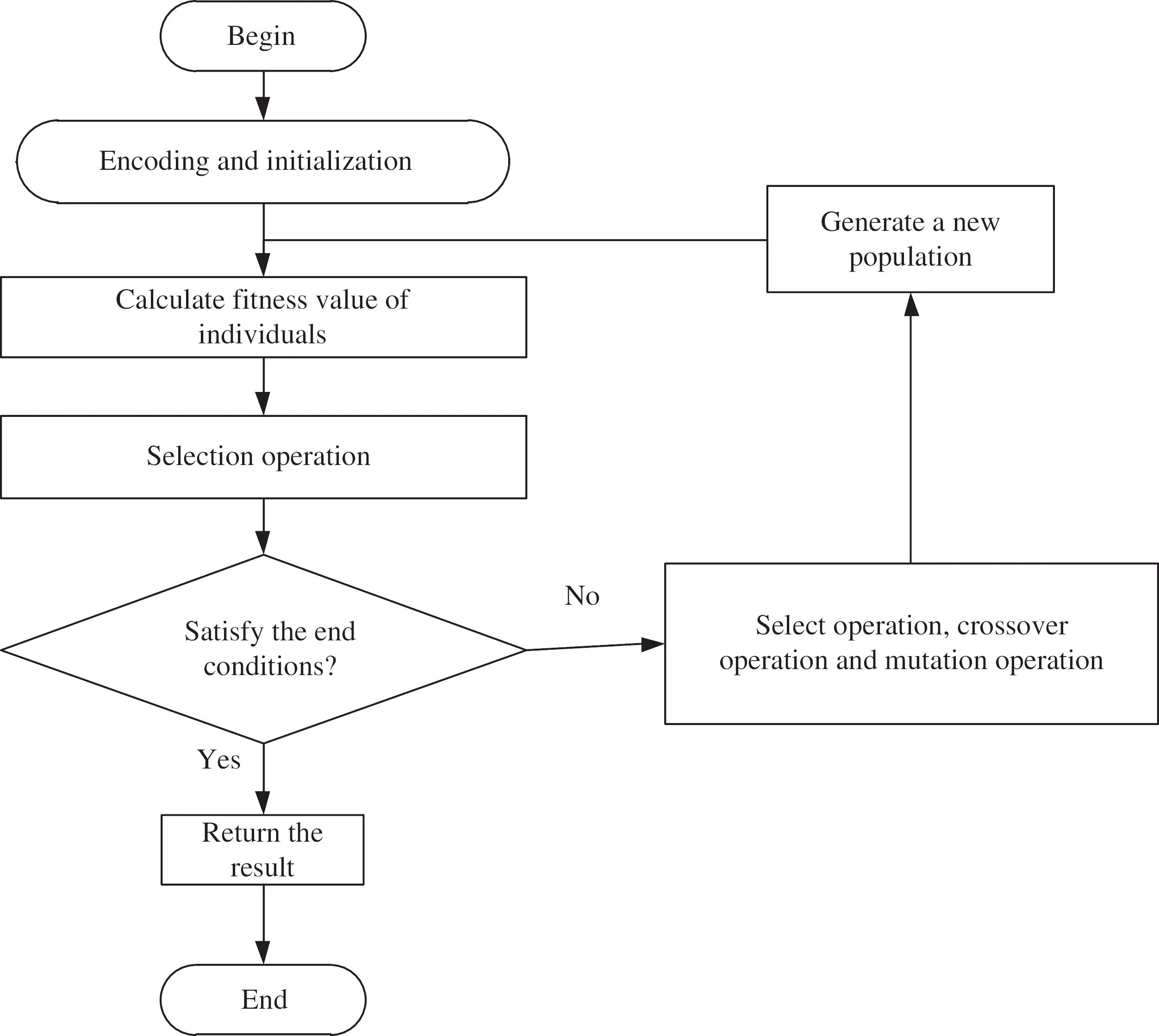Figure 3: The detailed flow of algorithm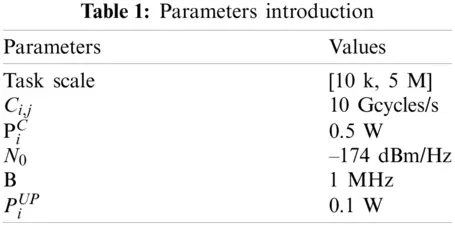In our experiment, we simulated four algorithms—MRR, ASS, DSS, and the GA-EC—On the performance of energy consumption with different task scales. When the task scale is small, the performance of ASS and MRR on energy consumption is not very good. The energy consumption of DSS is lower than that of MRR and ASS. However, the performance of GA-EC on energy consumption is the best, as shown in Fig. 4, and the advantage becomes more and more obvious as task scale increases, as shown in Fig. 5.Figure 4: Total energy consumption vs. task scale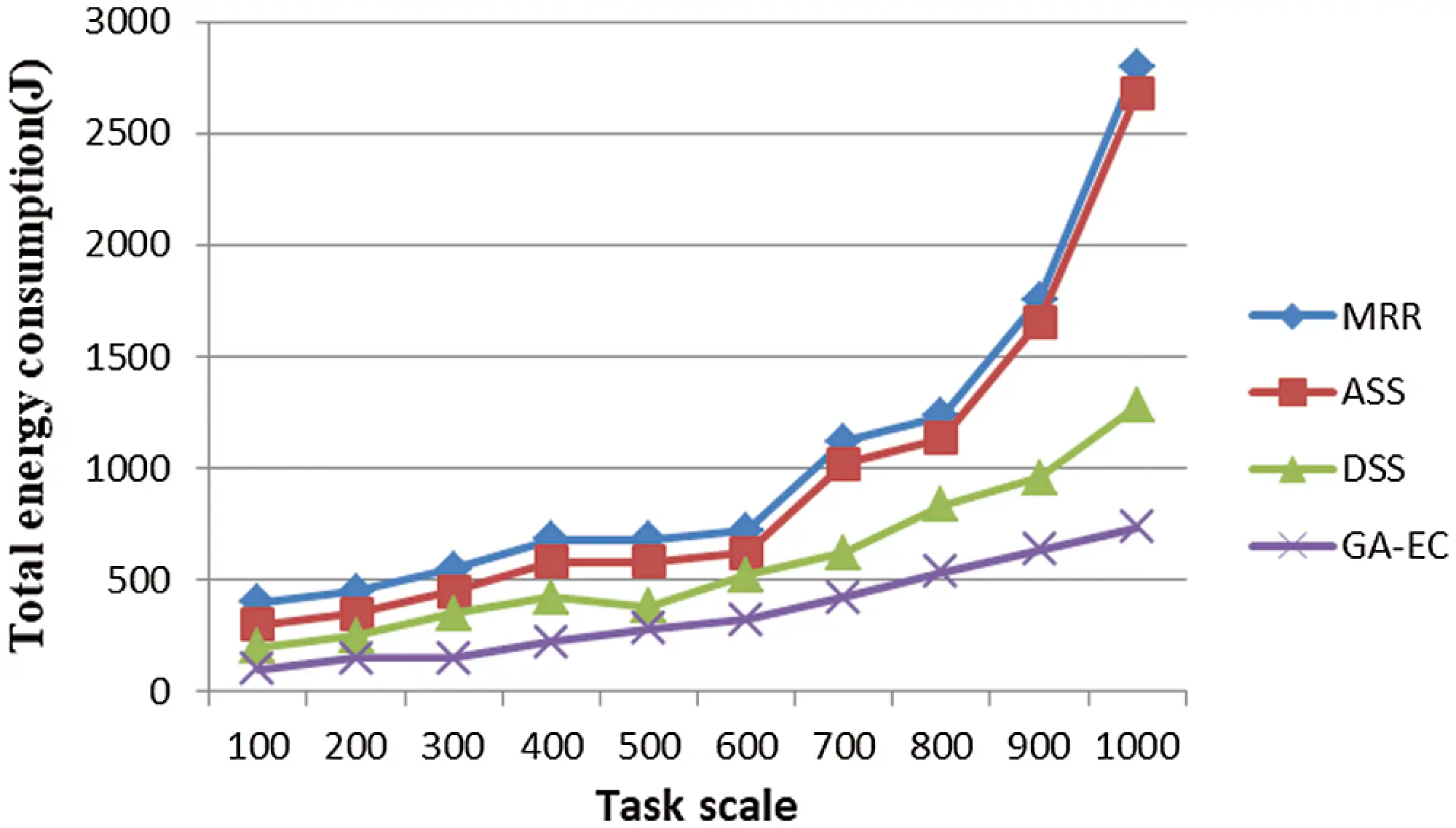Figure 5: Total energy consumption vs. task scale

Because time delay is such an important factor in the user experience, we simulated the performance of computing time delay and transmission time delay. We found that the computing time delay and transmission time delay grew longer as the number of tasks increased, as shown in Figs. 6 and 7. We also found that the performance of GA-EC on time delay was always better than other scheduling methods. Fig. 8 shows the simulation results of completion time with different task scales. We found that the advantage of the proposed algorithm GA-EC became more and more pronounced on the performance of total completion time as task scale increased.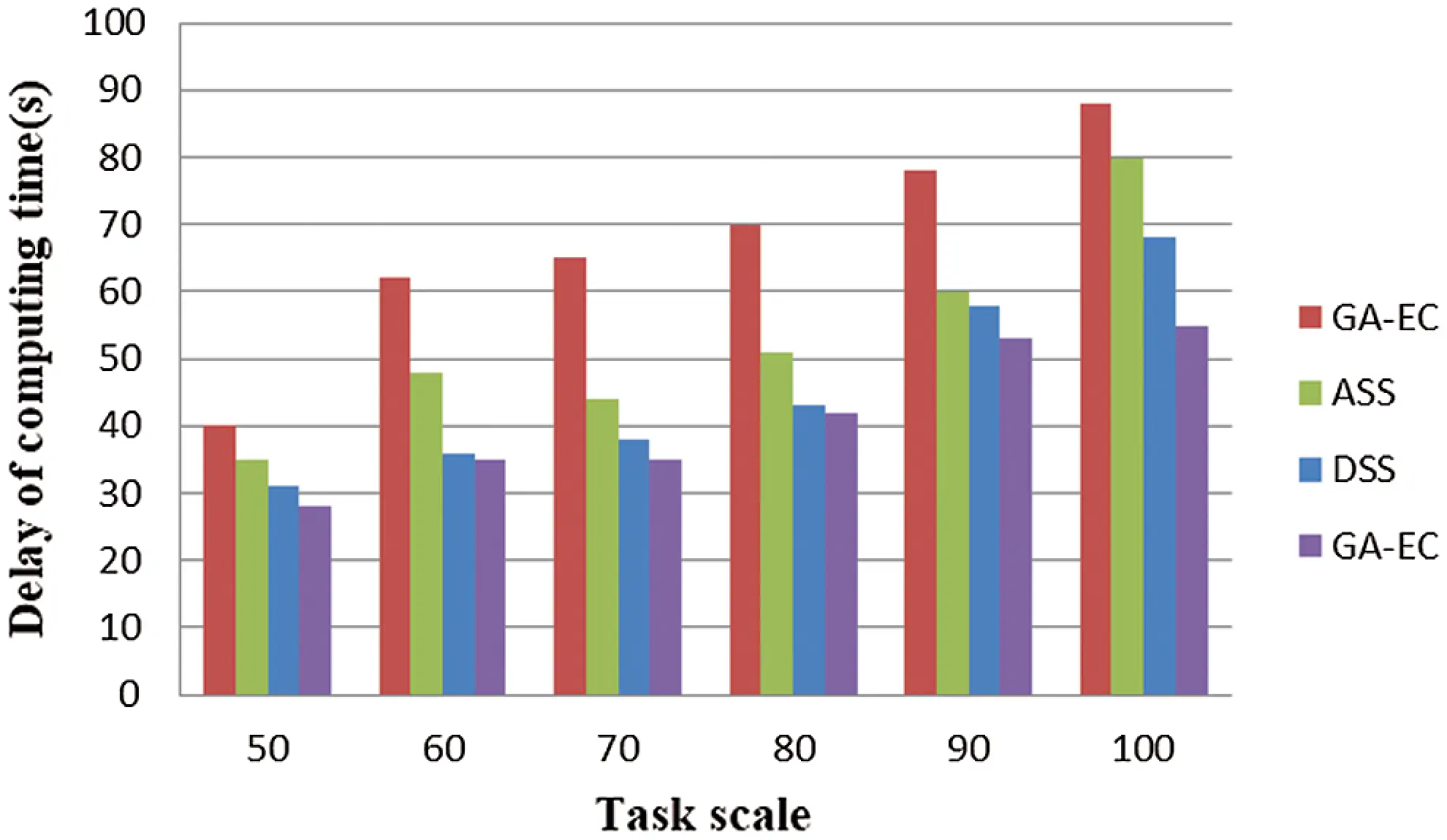Figure 6: Delay of computing time vs. Task scale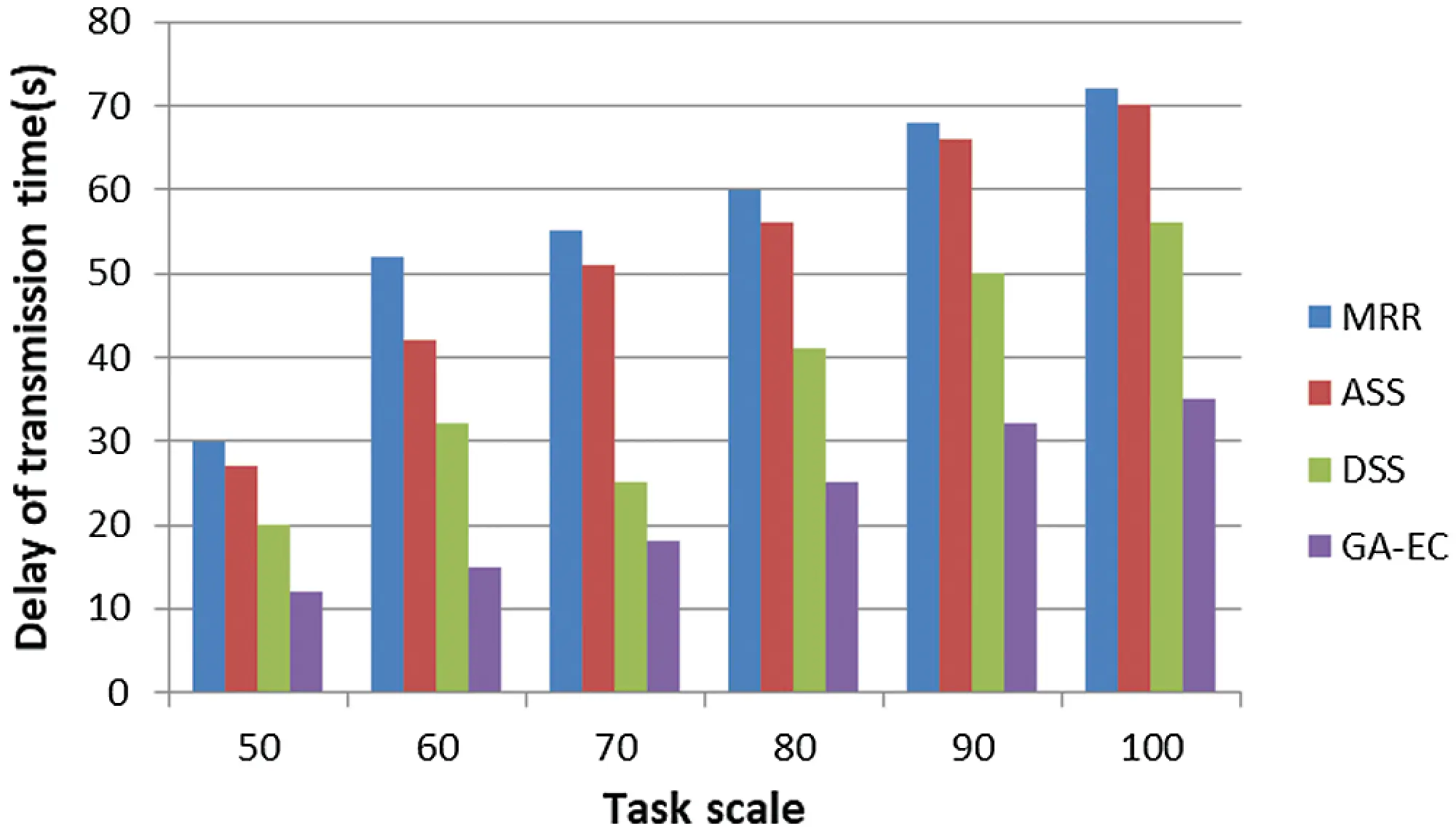Figure 7: Delay of transmission time vs. Task scale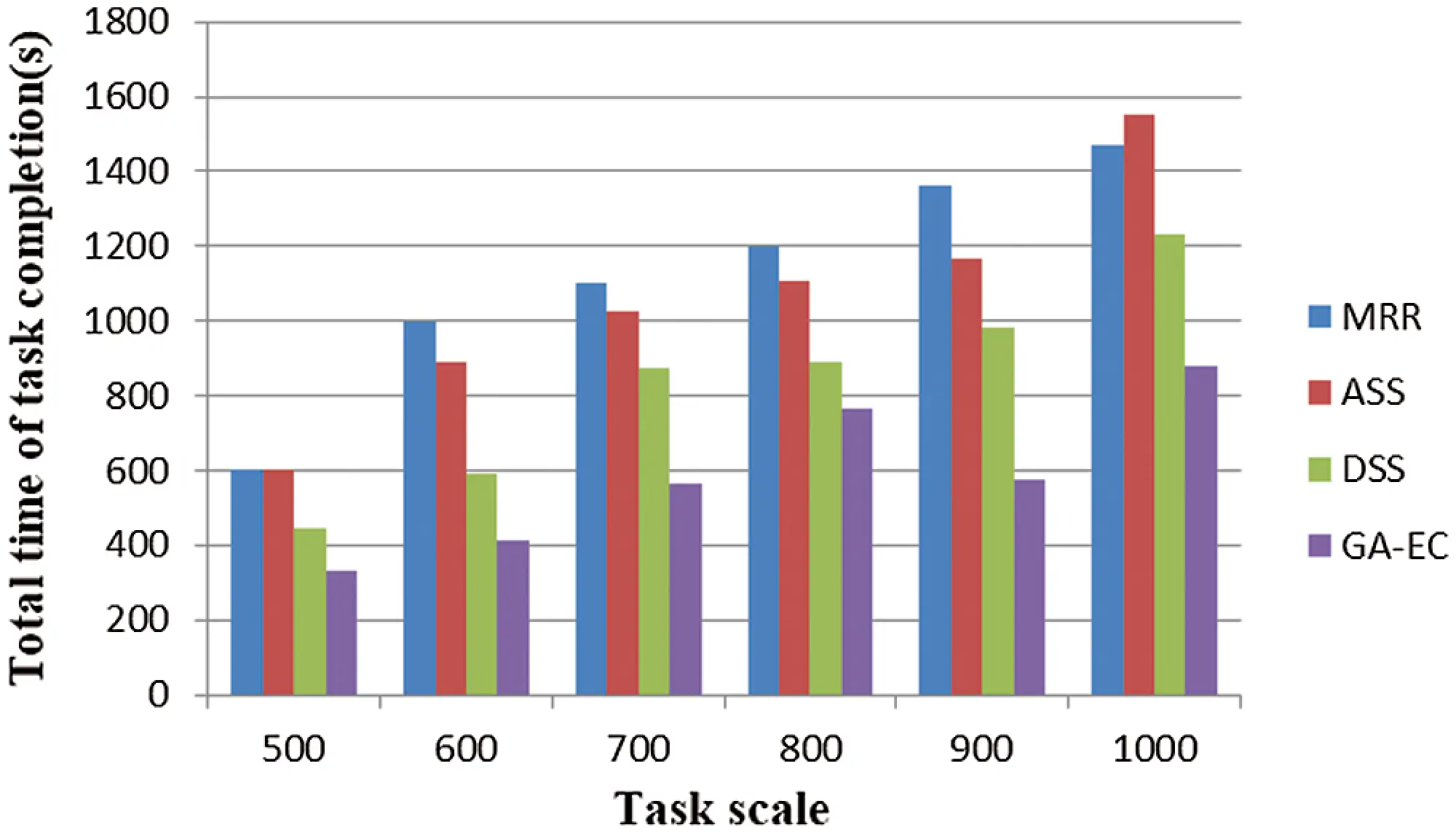The performance on load balance is also an important evaluation index for the task scheduling algorithm. Therefore, a new evaluation indicator is proposed, called the degree of imbalance. The degree of imbalance can be expressed as VDI=TmaxTminTavg, where Tmax, Tmin, and Tavg represent the longest time, the shortest time, and the average time of task completion. The load imbalance levels of different methods are shown in Fig. 9. We found that the degree of imbalance with GA-EC was always lower than that of the other scheduling methods. Therefore, compared to the ASS, DSS, and MRR algorithms, the GA-EC algorithm offers the best performance in terms of load balance.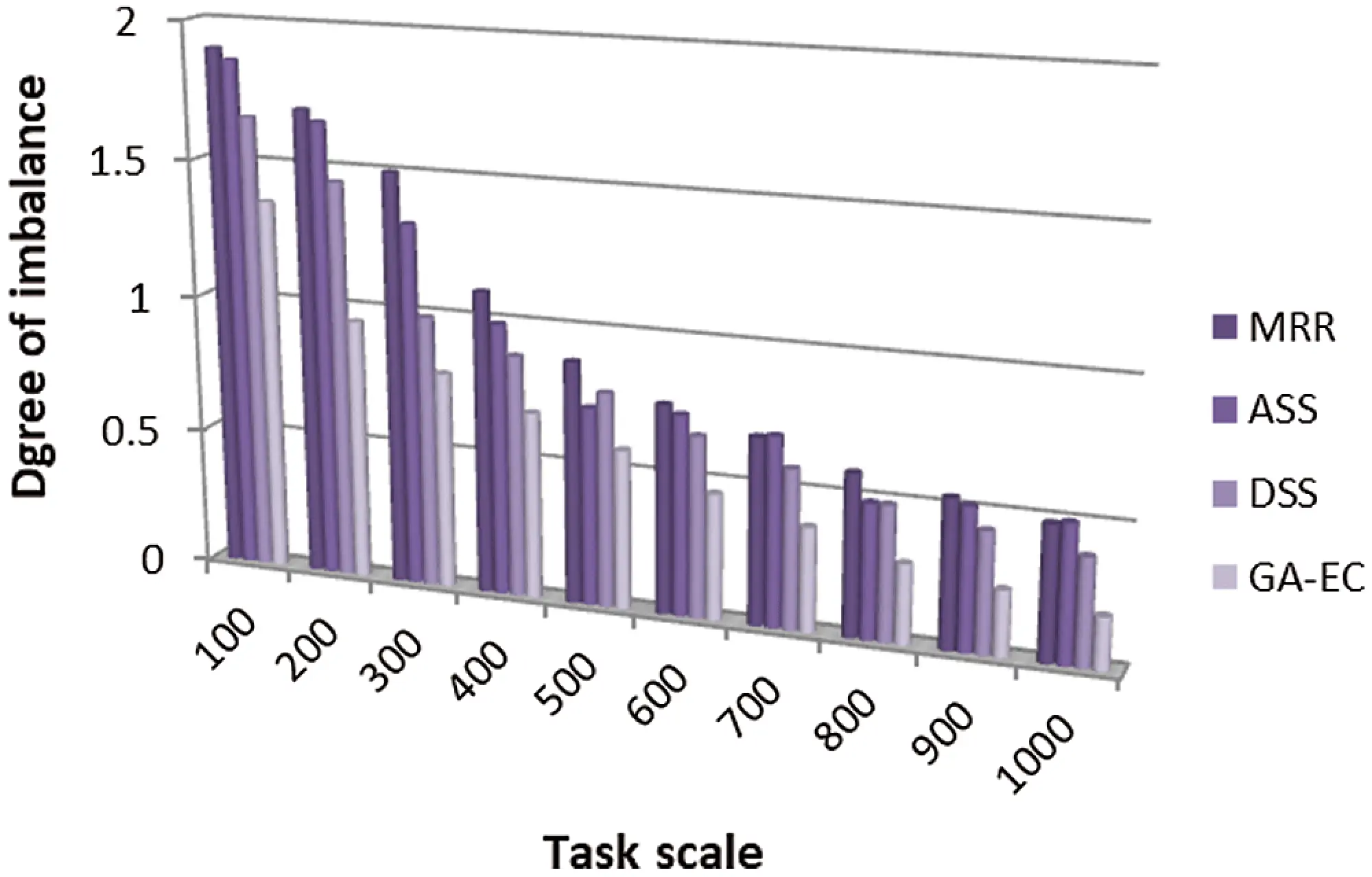Figure 9: Degree of imbalance vs. Task scale

5  Conclusion

To ensure the efficient and stable operation of the power cloud computing system as well as the effective use of edge computing nodes, a novel resource management model is proposed based on the concept of edge computing for the smart grid. For this model, computing tasks and data can be executed and stored in the local edge nodes. The resources of edge need to be shared because the resources in a single node are limited. The resource-intensive tasks can be divided into subtasks and then executed on different edge nodes. Therefore, a task scheduling algorithm based on a genetic algorithm is proposed to resolve the task scheduling problem. The simulation result shows that the proposed algorithm has a beneficial effect on energy consumption and load balancing, and reduces time delay. In the future, the authors will study other problems related to task scheduling, such as time delay and network bandwidth.

Funding Statement: This work was supported by the “National Key Research and Development Program of China” (No. 2020YFB0905900).

Conflicts of Interest: The authors declare that they have no conflicts of interest to report regarding the present study.

## References

1. M. Jia, X. Gu and Q. Guo, “Broadband hybrid satellite-terrestrial communication systems based on cognitive Radio toward 5G,” IEEE Wireless Communications, vol. 23, no. 6, pp. 96–106, 2016.
2. X. Liu, M. Jia, X. Y. Zhang and W. D. Lu, “A novel multichannel internet of things based on dynamic spectrum sharing in 5G communication,” IEEE Internet of Things Journal, vol. 6, no. 4, pp. 5962–5970, 2019.
3. M. Jia, Z. Yin and Q. Guo, “Downlink design for spectrum efficient IoT network,” IEEE Internet of Things Journal, vol. 5, no. 5, pp. 3397–3404, 2018.
4. A. Fox, R. Griffith and A. Joseph, “Above the clouds: A berkeley view of cloud computing,” Electrical Engineering and Computer Sciences, vol. 28, no. 13, pp. 3–5, 2009.
5. H. Hui, C. Zhou, S. Xu and F. Lin, “Research on resource management of power cloud system based on sinking computation,” Power System Technology, vol. 43, no. 12, pp. 4322–4328, 2019.
6. N. Zhang, X. L. Yang and M. Zhang, “Crowd-Funding: A new resource cooperation mode for mobile cloud computing,” PLOS One, vol. 11, no. 12, pp. e0167657, 201
7. H. Zhang, G. Chen and X. Li, “Resource management in cloud computing with optimal pricing policies,” Computer Systems Science and Engineering, vol. 34, no. 4, pp. 249–254, 2019.
8. L. Li, Y. Wei, L. Zhang and X. Wang, “Efficient virtual resource allocation in mobile edge networks based on machine learning,” Journal of Cyber Security, vol. 2, no. 3, pp. 141–150, 2020.
9. G. Laszewski, L. Wang, A. J. Younge and X. He, “Power-aware scheduling of virtual machines in DVFS-enabled clusters,” in Proc. IEEE Int. Conf. on Cluster Computing 2009, New Orleans, LA, USA, pp. 1–10, 200
10. H. Mi, H. Wang and G. Yin, “Resource on-demand reconfiguration method for virtualized data centers,” Journal of Software, vol. 22, no. 9, pp. 2193–2205, 2011.
11. B. Jo, M. J. Piran, D. Lee and D. Y. Suh, “Efficient computation offloading in mobile cloud computing for video streaming over 5g,” Computers, Materials & Continua, vol. 61, no. 2, pp. 439–463, 2019.
12. A. Y. Hamed, M. H. Alkinani and M. R. Hassan, “A genetic algorithm to solve capacity assignment problem in a flow network,” Computers, Materials & Continua, vol. 64, no. 3, pp. 1579–1586, 2020.
13. A. Y. Hamed, M. H. Alkinani and M. R. Hassan, “Ant colony optimization for multi-objective multicast routing,” Computers, Materials & Continua, vol. 63, no. 3, pp. 1159–1173, 2020.
14. M. Shojafar, N. Cordeschi and E. Baccarelli, “Energy-efficient adaptive resource management for real-time vehicular cloud services,” IEEE Transactions on Cloud Computing, vol. 7, no. 1, pp. 196–209, 2019.
15. L. Jiang and Z. Fu, “Privacy-preserving genetic algorithm outsourcing in cloud computing,” Journal of Cyber Security, vol. 2, no. 1, pp. 49–61, 2020.
16. T. Ma, S. Pang, W. Zhang and S. Hao, “Virtual machine based on genetic algorithm used in time and power oriented cloud computing task scheduling,” Intelligent Automation and Soft Computing, vol. 25, no. 3, pp. 605–614, 2019.
17. C. Gong, F. Lin and X. Gong, “Intelligent cooperative edge computing in internet of things,” IEEE Internet of Things Journal, vol. 7, no. 10, pp. 9372–9382, 2020.
18. Q. Y. Guo and F. D. Zhu, “Cloud computing resource scheduling algorithm based on ant colony algorithm and leap frog algorithm,” Bulle-Tin of Science and Technology, vol. 33, no. 5, pp. 167– 170, 2017.
19. H. Nie, “Optimization management of task scheduling for cloud resource load balance,” Computer Engineering and Design, vol. 38, no. 1, pp. 18–21, 2017.
20. D. M. Quan, F. Meezza and D. Sannenli, “T-Alloc: A practical energy efficient resource allocation algorithm for traditional data centers,” Future Generation Computer Systems, vol. 28, no. 5, pp. 791–800, 2012.
21. A. Beloglazov, J. Abawajy and R. Buyya, “Energy-aware resource allocation heuristics for efficient management of data centers for Cloud computing,” Future Generation Computer Systems, vol. 28, no. 5, pp. 755–768, 2012.
22. Y. F. Cui, X. M. Li and K. M. Dong, “Cloud computing resource dispatching method research based on improved genetic algorithm,” Advanced Materials Research, vol. 271, no. 4, pp. 552–557, 2011.
23. J. Gu, J. Hu and T. Zhao, “A new resource dispatching strategy based on genetic algorithm in cloud computing environment,” Journal of Computer, vol. 7, no. 1, pp. 42–52, 2012.
24. Y. Sun, F. H. Lin and H. T. Xu, “Multi-objective optimization of resource scheduling in fog computing using an improved NSGA-II,” Wireless Personal Communications, vol. 102, no. 2, pp. 1369–1385, 2018.
25. N. Zhang, X. L. Yang and M. Zhang, “A genetic algorithm-based task scheduling for cloud resource crowd-funding model,” Int. Journal of Communication Systems, vol. 31, no. 1, pp. 1–10, 2017.
26. P. Pradhan, P. K. Behera and B. N. B. Ray, “Modified round robin algorithm for resource allocation in cloud computing,” Procedia Computer Science, vol. 85, no. 9, pp. 878–890, 2016.
27. X. Chen, “Decentralized computation offloading game for mobile cloud computing,” IEEE Transactions on Parallel and Distributed Systems, vol. 26, no. 4, pp. 974–983, 2015.This work is licensed under a Creative Commons Attribution 4.0 International License, which permits unrestricted use, distribution, and reproduction in any medium, provided the original work is properly cited.